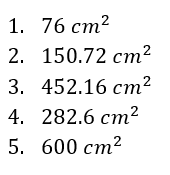# Surface area worksheet (with answer key)

An object’s surface area is the total area that all of its surfaces occupy. The formulas we will learn in this post make it simple to determine the various surface areas that various 3D shapes in geometry have.

## What is the “Surface area worksheet”?

The surface area worksheet is a math practice sheet that incorporates application, reflection, assessment, word problems, and problem-solving with a challenge to aid students in creating and resolving their word problems. This activity will assist students in understanding the lecture, applying new knowledge, and utilizing prior knowledge.

## Instructions on how to use the “Surface area worksheet.”

Use this math worksheet to explore the surface area in detail and learn how to compute them. We provide a 5-item activity to help you practice the topic.

.

The worksheet’s last part, titled “Reflection,” invites students to assess their learning throughout the lecture and their metacognition.

### Conclusion

Computing the surface area is simple as long as you remember the steps and are familiar with the formula.

Surface area worksheet (with answer key)

## Notes to remember:

The following are the formulas for the following shapes:

• Cuboid
• Cube
• Cone
• Cylinder
• Pyramid
• Sphere

## Step-by-step Example:

Find the surface area of a cube whose length of the sides is 4 cm each.

Step 1:

To solve for the surface area, use the formula:

Step 2:

Solve for the surface area.

## Surface area worksheet (with answers)

Application:

Solve the following and show your solutions in the box provided:

1. Find the surface area of a cuboid whose length is 2 cm, width is 5 cm, and height is 4 cm.1. Find the surface area of a cone whose radius is 4 cm and has a height of 8 cm.1. Find the surface area of a sphere whose radius is 6 cm.1. Find the surface area of a cylinder whose radius is 3 cm and height is 12 cm.1. Find the surface area of a cube whose sides are 10 cm each.Reflection

What do you think about solving the surface area? Did you find it difficult to follow the pattern in each step?.